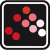Scilab
Operating system: Windows
Category: Education
Review rating:
Official page: Scilab
Wikipedia: Scilab

#### Description

Scilab – a software to perform the complex engineering and mathematical tasks. The software contains many mathematical functions to solve the algebraic and geometric equations. Scilab is able to work with the 2D and 3D graphics, integrals, matrices, polynomials, differential equations, etc. The software allows you to add the new mathematical functions that are written in the different programming languages. Scilab contains a special module which enables to simulate the trajectory of the bike or attraction of a black hole by the specified parameters.

#### Main features:

• A large number of the mathematical functions
• 2D and 3D visualization
• Data analysis
• Modelling of the mechanical and hydraulic systems
• Signal processingVersion:
6.1
Architecture:
Language:
English